# WBJEE 2018 Maths Paper with Solutions

WBJEE 2018 Maths question paper with solutions is a perfect resource for those who are preparing for WBJEE examination. The first step towards the WBJEE exam preparation is to solve WBJEE Previous Year Question Papers. Along with the question paper, the answer key of WBJEE 2018 has also been provided here. Solving WBJEE previous year papers will give students an idea about the nature and pattern of questions asked in the exam. It will also help the students to get a clear idea about the amount of preparation required to face the exam.

### WBJEE 2018 - Maths

Question 1: If (2 ≤ r ≤ n), then nCr + 2 . nCr+1 + nCr+2 is equal to

1. a. 2. nCr+2
2. b. n+1Cr+1
3. c. n+2Cr+2
4. d. n+1Cr

Solution:

nCr + 2. nCr+1 + nCr+2

=nCr + nCr+1+ nCr+1 + nCr+2

=n+1Cr+1 + n+1Cr+2

=n+2Cr + 2

Question 2: The number (101)100 – 1 is divisible by

1. a. 104
2. b. 106
3. c. 108
4. d. 1012

Solution:

(101)100 – 1 =(1 + 100)2 –1

=[100C0 +100C1 100 + 100C2 (100)2+ ………+ 100C100(100)100 (100C0)=1

(1+100)100-1= 1 + 100(100)+100C21002+………+100C100100100-1

=1002(1+ 100C2 +100C3(100)+………+100C10010098)

So, 101100-1 is divisible by 104

Question 3: If n is even positive integer, then the condition that the greatest term in the expansion of (i + x)n may also have the greatest coefficient is

1. a. n / [n + 2] < x < [n + 2] / n
2. b. n / [n + 1] < x < [n + 1] / n
3. c. [n + 1] / [n + 2] < x < [n + 2] / [n + 1]
4. d. [n + 2] / [n + 3] < x < [n + 3] / [n + 2]

Solution:

For the greatest term, we have

n / 2 < {[n + 1] / 1 + | x |} ≤ (n / 2) + 1

n / 2 < {[n + 1] / 1 + | x |} and [n + 1] / | x | + 1 + ≤ (n / 2) + 1

[1 + | x |] / 2 + < [n + 1] / n + and [n + 1] / [n + 2] ≤ [| x | + 1] / 2

|x| < {[2n + 2] / [n]} - 1 and [2n + 2] / [n + 2] –1 ≤ |x|

x < [n + 2] / n and [n] / [n + 2] ≤ x

[n] / [n + 2] < x [n + 2] / n

Question 4: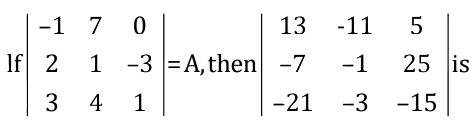1. a. A2
2. b. A2 – A + I3
3. c. A2 – 3A + I3
4. d. 3A2 + 5A – 4I3

Solution:

Given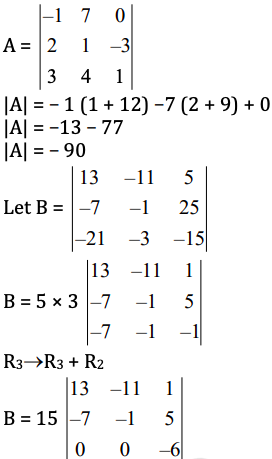B = 15 [0 – 0 – 6 (–90)]

B = (–90) (–90)

B = A. A

B = A2

Question 5: If ar = (cos2r𝛑 + i sin 2r𝛑)1/9, then the value of

$$\begin{vmatrix} a_1 &a_2 &a_3 \\ a_4 & a_5 & a_6\\ a_7 & a_8 &a_9 \end{vmatrix}$$
is

1. a. 1
2. b. –1
3. c. 0
4. d. 2

Solution:

Given ar = (cos2r𝛑 + i sin 2r𝛑)1/9

ar = e2r𝛑i/9

Now,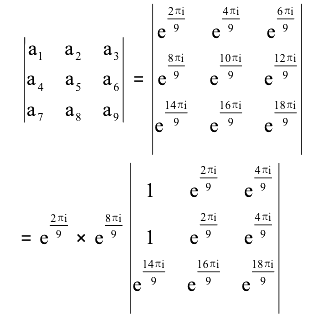= 0 {two rows are same}

Question 6: If Sr =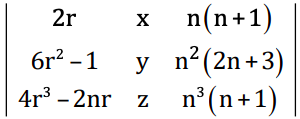Then the value of ∑r=1n Sr is independent of

1. a. x only
2. b. y only
3. c. n only
4. d. x, y, z and n

Solution: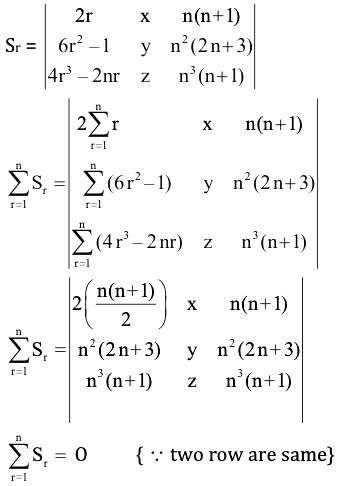Question 7: If the following three linear equations have a non –trivial solution, then x + 4ay + az = 0, x + 3by + bz = 0, x + 2cy + cz = 0

1. a. a, b, c are in A.P
2. b. a, b, c are in G.P.
3. c. a, b, c are in H.P
4. d. a + b + c = 0

Solution:

System equations

x + 4ay + az = 0

x + 3by + bz = 0

x + 2cy + cz = 0

For non-trivial solution

Δ = 0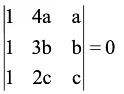1(3bc – 2bc) – 1 (4ac – 2ac) + (4ab – 3 ab) = 0

bc – 2ac + ab = 0

bc + ab = 2ac

b = 2ac / a + c

a, b, c are in H.P.

Question 8: On R, a relation 𝞀 is defined by x𝞀y if and only if x–y is zero or irrational. Then

1. a. 𝞀 is equivalence relation
2. b. 𝞀 is reflexive but neither symmetric nor transitive
3. c. 𝞀 is reflexive & symmetric but not transitive
4. d. 𝞀 is symmetric & transitive but not reflexive

Solution:

xRy ⇒ x – y is zero or irrational

xRx ⇒ 0 [hence reflexive]

ifxRy ⇒ x – y is zero or irrational

⇒ y – x is zero or irrational

yRx symmetric

xRy ⇒ x – y is 0 or irrational

yRz ⇒ y – z is 0 or irrational

Then (x – y) + (y – z) = x – z may be rational

It is not transitive.

Question 9: On the set R of real numbers, the relation 𝞀 is defined by x𝞀y,(x,y) ∈ R.

1. a. if |x – y| < 2 then 𝞀 is reflexive but neither symmetric nor transitive
2. b. if x – y < 2 then 𝞀 is reflexive and symmetric but not transitive
3. c. if |x| ≥ y then 𝞀 is reflexive and transitive but not symmetric
4. d. if x > |y| then 𝞀 is transitive but neither reflexive nor symmetric

Solution:

(x, x) ∈ R ⇒ x > |x| {false}

not reflexive

If (x, y) ∈ R ⇒ x > |y| ⇒ y > |x|

not symmetric

If (x, y) ∈ R ⇒ x > |y|; (y, z) ∈ R y > |z|

x > |z| ⇒ (x, z) ∈ R

Transitive

Question 10: If f : R → R be defined by f (x) = ex and g : R →R be defined by g (x) = x2. The mapping g of : R → R be defined by (g of )x = g [f (x)] ∀ x ∈ R, then

1. a. gof is bijective but f is not injective
2. b. gof is injective and g is injective
3. c. gof is injective but g is not bijective
4. d. gof is surjective and g is surjective

Solution:

Given f (x) = ex

g (x) = x2

(g of) (x) = g [f (x)]

= g [ex]

= (ex)2

= e2x : x ∈ R

Clearly g(f(x)) is injective but g(x) is not injective.

Question 11: In order to get heads at least once with probability ≥ 0.9, the minimum number of times an unbiased coin needs to be tossed is

1. a. 3
2. b. 4
3. c. 5
4. d. 6

Solution:

p (H) = 1 / 2

p (T) = 1 / 2

P = 1 - (1 / 2n) ≥ 0.9

1 - (9 / 10) ≥ (1 / 2n)

(1 / 10) ≥ (1 / 2n)

10 ≤ 2n

n = 4

Question 12: A student appears for tests I, II and III. The student is successful if he passes in tests I, II or III. The probabilities of the student passing in tests I, II and III are respectively p,q and 1 / 2. If the probability of the student to be successful is 1 / 2. Then

1. a. p (1 + q) = 1
2. b. q (1 + p) =1
3. c. pq = 1
4. d. (1 / p) + (1 / q) = 1

Solution:

Let A, B and C be the events that the student is successful in tests I, II and III respectively. Then p (The student is successful)

= P [(I ∩ II ∩ III’) ⋃ (I ∩ II’ ∩ III) ⋃ (I ∩ II ∩ III)]

1 / 2 = P (I ∩ II ∩ III’) + P (I ∩ II’ ∩ III) + P (I ∩ II ∩ III)

1 / 2 = P (I) P (II) P (III’) + P (I) P (II’) P (III) + P (I) P (II) P (III) [I, II and III are independent]

1 / 2 = p . q (1 - [1 / 2]) + p . (1 - q) . (1 / 2) + p . q . (1 / 2)

1 = pq + p

p (q + 1) = 1

Now,

⇒ P2 = q

⇒ (a)2 = 2a

⇒ a = 2

Question 13: If sin 6θ + sin4θ + sin2θ = 0, then general value of θ is (n is integer)

1. a. n𝛑 / 4, n𝛑 ± (𝛑 / 3)
2. b. n𝛑 / 4, 4𝛑 ± (𝛑 / 6)
3. c. n𝛑 / 4, 2n𝛑 ± (𝛑 / 3)
4. d. n𝛑 / 4, 2n𝛑 ± (𝛑 / 6)

Solution:

sin 6θ + sin4θ + sin2θ = 0

sin 4θ + 2 sin (8θ / 2) cos (4θ / 2) = 0

sin 4θ + 2 sin 4θ cos 2θ = 0

sin 4θ (1 + 2 cos 2θ) = 0

sin 4θ = 0 or 1 + 2 cos 2θ = 0

4θ = n𝛑 or cos 2θ = -1 / 2 = cos (2𝛑 / 3)

θ = n𝛑 / 4 or 2θ = 2n𝛑 ± (2𝛑 / 3)

θ = n𝛑 / 4 or θ = n𝛑 ± (𝛑 / 3)

Question 14: If 0 ≤ A ≤ 𝛑 / 4, then tan–1 (1 / 2) tan 2A + tan–1 (cot A) + tan–1 (cot3A) is equal to

1. a. 𝛑 / 4
2. b. 𝛑
3. c. 0
4. d. 𝛑 / 2

Solution:

tan–1 (1 / 2) tan 2A + tan–1 (cot A) + tan–1 (cot3A)

= tan–1 (1 / 2) tan 2A + tan-1 [(cot A + cot3 A) / (1 - cot4 A)]

= tan-1 [(1 / 2) [2 tan A / (1 - tan2 A)]] + tan-1 [cot A / (1 - cot2 A)]

= tan-1 [tan A / (1 - tan2 A)] + tan-1 [tan A / (tan2 A - 1)]

= tan-1 [tan A / (1 - tan2 A)] - tan-1 [tan A / (1 - tan2 A)]

= 0

Question 15: Without changing the direction of the axes, the origin is transferred to the point (2, 3). Then the equation x2 + y2 – 4x – 6y + 9 = 0 changes to

1. a. x2 + y2 + 4 = 0
2. b. x2 + y2 = 4
3. c. x2 + y2 – 8x – 12y + 48 = 0
4. d. x2 + y2 = 9

Solution:

By replacing

X → x + 2, y → y + 3

Given equation of circle is x2 + y2 – 4x – 6y + 9 = 0

(x + 2)2 + (y + 3)2 – 4 (x + 2) – 6 (y + 3) + 9 = 0

x2 + 4x + 4 + y2 + 6y + 9 – 4x – 8 – 6y – 18 + 9 = 0

x2 + y2 – 4 = 0

Question 16: The angle between a pair of tangents drawn from a point P to the circle x2 + y2 + 4x – 6y + 9sin2α + 13cos2α = 0 is 2α. The equation of the locus of the point P is

1. a. x2 + y2 + 4x + 6y + 9 = 0
2. b. x2 + y2 – 4x + 6y + 9 = 0
3. c. x2 + y2 – 4x – 6y + 9 = 0
4. d. x2 + y2 + 4x – 6y + 9 = 0

Solution:

Let the centre be O, points on circle from where tangents are drawn is A, B and point of intersection of tangent is P.

x2 + y2 + 4x – 6y + 9sin2α + 13cos2α = 0

Centre of circle O = (–2, 3)

r = √(4 + 9 - 9 sin2 α - 13 cos2 α)

= √(13 - 9 sin2 α - 13 (1 - sin2 α))

= √(13 sin2 α - 9 sin2 α)

= 2 sin α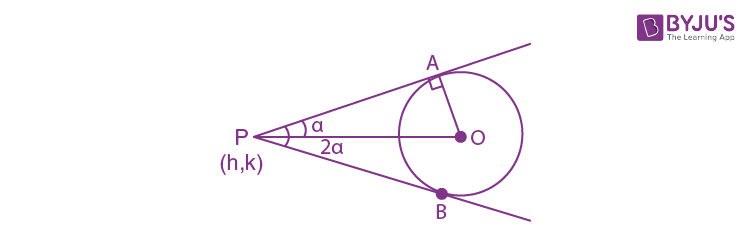2α is the angle between tangents

sin x = OA / OP

sin x = 2 sinx / √(h + 2)2 + (k - 3)2

(h + 2)2 + (k – 3)2 = 4

h2 + k2 + 4h – 6k + 9 = 0

Focus of point p is

x2 + y2 + 4x – 6y + 9 = 0

Question 17: The point Q is the image of the point P (1, 5) about the line y = x and R is the image of the point Q about the line y = -x. The circumcentre of the PQR is

1. a. (5, 1)
2. b. (–5, 1)
3. c. (1, –5)
4. d. (0, 0)

Solution:

Clearly P (1, 5)

Q = (5, 1) {as y = x}

R = (–1, –5) {as y = –x}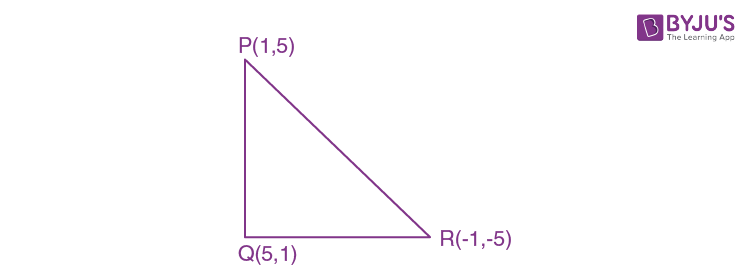Circum center of PQR is (1 – 1) / 2, (5 – 5) / 2 = (0, 0)

Question 18: The angular points of a triangle are A (–1, –7), B (5, 1) and C (1, 4). The equation of the bisector of the angle ∠ABC is

1. a. x = 7y + 2
2. b. 7y = x + 2
3. c. y = 7x + 2
4. d. 7x = y + 2

Solution: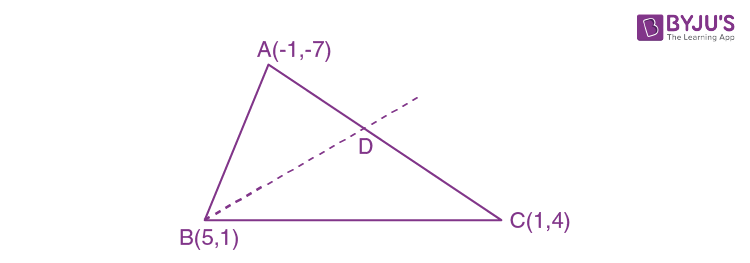AB = √(- 1 - 5)2 + (- 7 - 1)2 = 10

BC = √(1 - 5)2 + (4 - 1)2 = 5

BD divides AC in ratio 2 : 1

D = [(-1 + 2) / (2 +1), (-7 + 8) / (2 + 1)]

= (1 / 3, 1 / 3)

Equation of BD is

y - 1 = [(1 - (1 / 3)) / (5 - (1 / 3))] (x - 5)

y - 1 = (2 / 14) (x - 5)

x – 7 y + 2 = 0

7y = x + 2

Question 19: If one the diameters of the circle, given by the equation x2 + y2 + 4x + 6y – 12 = 0, is a chord of a circle S, whose centre is (2, –3), the radius of S is

1. a. √41 unit
2. b. 3 √5 unit
3. c. 5 √2 unit
4. d. 2 √5 unit

Solution:

Given: equation of circle is x2 + y2 + 4x + 6y – 12 = 0.

Whose centre is (2, –3) and radius = √22 + (–3)2 + 12 = 5

Now, according to the given information, we have the following figure.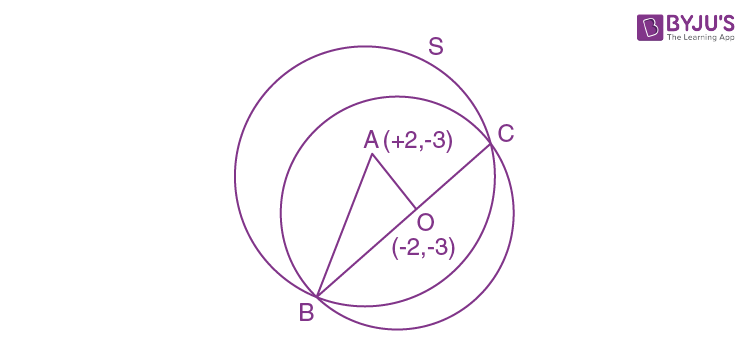x2 + y2 + 4x + 6y – 12 = 0

Clearly, AO ⊥ BC, as O is mid point of the chord.

Now, in △AOB we have

OA = √[(2 + 2)2 + (-3 + 3)2] = √16 = 4 and OB = 5

AB = √[OA2 + OB2]

= √[16 + 25]

= √41

Question 20: A chord AB is drawn from point A (0, 3) on the circle x2 + 4x + (y – 3)2 = 0, and is extended to M such that AM = 2AB. The locus of M is

1. a. x2 + y2 – 8x – 6y + 9 = 0
2. b. x2 + y2 + 8x + 6y + 9 = 0
3. c. x2 + y2 + 8x – 6y + 9 = 0
4. d. x2 + y2 – 8x + 6y + 9 = 0

Solution:

Given equation of circle is x2 + 4x + (y – 3)2 = 0

AM = 2AB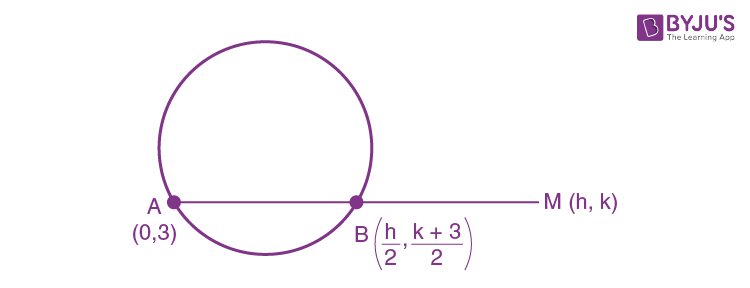B is the mid point of AM

B = [(h / 2), (k + 3) / 2] lies on the circle.

Equation of circle is x2 + 4x + (y – 3)2 = 0

Let x = h / 2, y = (k + 3) / 2

h2 / 4 + 2h + [{(k + 3) / 2} - 3]2 = 0

(h2 / 4) + 2h + [(k2 - 6k + 9) / 4] = 0

k2 + h2 + 8h – 6k + 9 = 0

Locus of m is x2 + y2 + 8x – 6y + 9 = 0.

Question 21: Let the eccentricity of the hyperbola x2 / a2 - y2 / b2 = 1 be reciprocal to that of the ellipse x2 + 9y2 = 9, then the ratio a2 : b2 equals

1. a. 8 : 1
2. b. 1 : 8
3. c. 9 : 1
4. d. 1 : 9

Solution:

Hyperbola: x2 / a2 - y2 / b2 = 1

Ellipse: x2 + 9y2 = 9

x2 / 9 + y2 / 1 = 1

The eccentricity of ellipse e = √[1 - (1/9)] = √(8/9)

The eccentricity of the hyperbola be reciprocal to the ellipse

The eccentricity of hyperbola = √(9/8)

1 + (b2 / a2) = 9 / 8

(b2 / a2) = 1 / 8

a2 : b2 = 8 : 1

Question 22: Let A, B be two distinct points on the parabola y2 = 4x. If the axis of the parabola touches a circle of radius r having AB as diameter, the slope of the line AB is

1. a. -1 / r
2. b. 1 / r
3. c. 2 / r
4. d. -2 / r

Solution: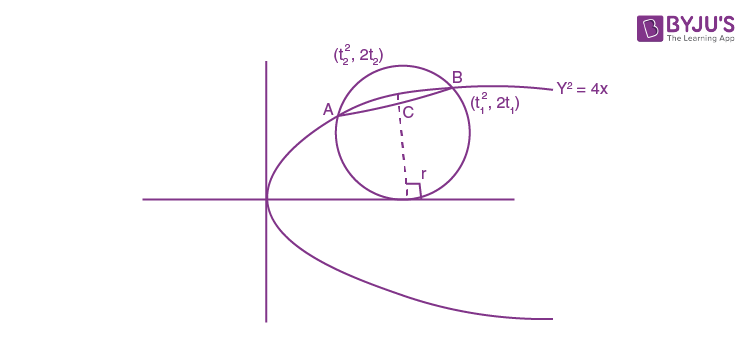Let, A (t22, 2t2), B (t12, 2t1) be the two points on the parabola.

AB is the diameter of the circle.

Let, c be the centre of the circle.

C = ([t12 + t22] / 2, t1 + t2)

This axis of the parabola is the x-axis.

So, the circle touches the x-axis.

Hence, distance of c from x-axis = radius of circle.

|t1 + t2| = r

(t1 + t2) = ± r

Slope of AB = [y2 - y1] / [x2 - x1]

= [2t1 - 2t2] / [t12 - t22]

= 2 / [t1 + t2]

= ± 2 / r

Question 23: Let P (at2, 2at), Q (ar2, 2ar) be three points on a parabola y2 = 4ax. If PQ is the focal chord and PK, QR are parallel where the co-ordinates of K is (2a, 0), then the value of r is

1. a. t / (1 - t2)
2. b. (1 - t2) / t
3. c. (t2 + 1) / t
4. d. (t2 - 1) / t

Solution: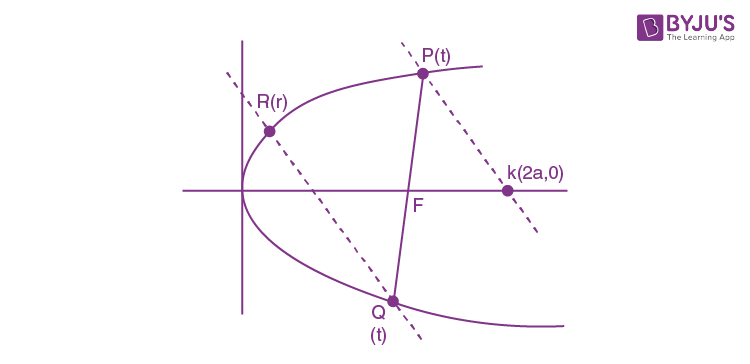The slope of line Pk = slope of line QR

mPk = mQR

[2at - 0] / [at2 - 2a] = [2at’ - 2ar] / [a (t’)2 - ar2]

t / [t2 - 2] = [t’ - r] / [(t’)2 - r2]

–t ' – tr2 = –t – rt2 – 2t ' + 2r {tt ' = –1}

t ' – tr2 = – t + 2r – rt2

–tr2 + r (t2 – 2) + t ' + t = 0

λ = (2 - t2) ± √(t2 - 2)2 + 4 (-1 + t2) / -2t

= (2 - t2) ± √t4 / [-2t]

= [(2 - t2) ± t2] / -2t

r = -1 / t

It is not possible as the R & Q will be one and same.

Or r = [t2 - 1] / 2

Question 24: Let P be a point on the ellipse x2 / 9 + y2 / 4 = 1 and the line through P parallel to the y-axis meet the circle x2 + y2 = 9 at Q, where P, Q are on the same side of the x-axis. If R is a point on PQ such that PR / RQ = 1 / 2 then the locus of R is

1. a. x2 / 9 + 9y2 / 49 = 1
2. b. x2 / 49 + y2 / 9 = 1
3. c. x2 / 9 + y2 / 49 = 1
4. d. 9x2 / 9 + y2 / 49 = 1

Solution:

Ellipse: x2 / 9 + y2 / 4 = 1

P = (3cosθ, 2sinθ)

Circle: x2 + y2 = 9

Q = (3cosθ, 3sinθ)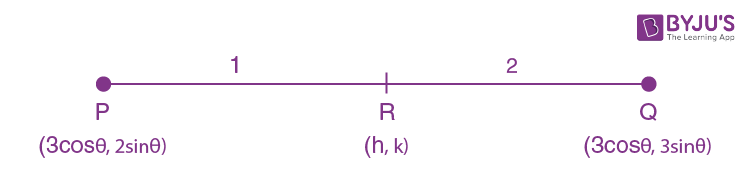h = [3 cos θ + 6 cos θ] / 3; k = [3 sin θ + 4 sin θ] / 3

h = 3 cos θ; k = (7 / 3) sin θ

sin2 θ + cos2 θ = 1

h2 / 9 + 9k2 / 49 = 1

The locus is (x2 / 9) + (9y2 / 49) = 1

Question 25: A point P lies on a line through Q (1, –2, 3) and is parallel to the line x / 1 = y / 4 = z / 5. If P lies on the plane 2x + 3y – 4z + 22 = 0, then segment PQ equals to

1. a. √42 units
2. b. √32 units
3. c. 4 unit
4. d. 5 units

Solution:

Equation line: (x - 1) / 1 = (y + 2) / 4 = (z - 3) / 5 = λ (let)

Point P (λ + 1, 4λ – 2, 5λ + 3)

Point p lies on 2x + 3y – 4z + 22 = 0

2(λ + 1) + 3 (4λ – 2) – 4(5λ + 3) + 22 = 0

–6λ + 6 = 0

λ = 1

Point p = (2, 2, 8), q = (1, –2, 3)

Distance PQ = √[12 + 42 + 52]

= √[1 + 16 + 25]

= √42

Question 26: The foot of the perpendicular drawn from the point (1, 8, 4) on the line joining the points (0, –11, 4) and (2, – 3, 1) is

1. a. (4, 5, 2)
2. b. (–4, 5, 2)
3. c. (4, –5, 2)
4. d. (4, 5, –2)

Solution:

Equation of line joining point (0, –11, 4) and (2, –3, 1)

(x - 2) / 2 = (y + 3) / 8 = (z - 1) / -3 = λ (let)

DR’s of PQ (2λ + 1, 8λ – 11, –3λ – 3)

Now, (2λ + 1) 2 + (8λ – 11)8 +(–3λ – 3)(–3) = 0

77λ - 77 = 0

λ = 1

Q = (4, 5, –2)

Question 27: The approximate value of sin31o is

1. a. > 0.5
2. b. > 0.6
3. c. < 0.5
4. d. < 0.4

Solution:

sin 30o = 1/2

sin 31o > 1/2 {since sinx is increasing function}

Question 28: Let f1(x) = ex, f2(x) = ef1(x), ............, fn+1(x) = efn(x) for all n ≥ 1. Then for any fixed n, (d/dx) fn(x): is

1. a. fn(x)
2. b. fn(x)fn–1(x)
3. c. fn(x)fn–1(x)....f1(x)
4. d. fn(x)......... f1(x)ex

Solution: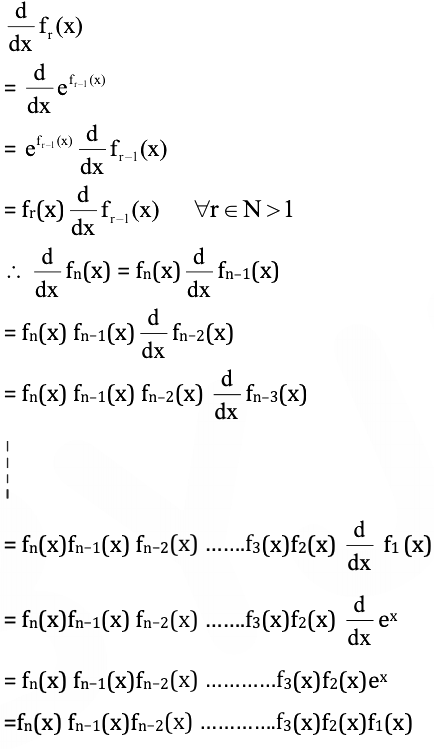Question 29: The domain of definition of f (x) = √[1 - |x|] / [2 - |x|] is

1. a. (–∞,–1) ⋃ (2, ∞)
2. b. [–1,1] ⋃ (2, ∞) ⋃ (–∞, – 2)
3. c. (–∞,1) ⋃ (2, ∞)
4. d. [–1,1] ⋃ (2, ∞)

Solution:

f (x) = √[1 - |x|] / [2 - |x|]

[1 - |x|] / [2 - |x|] ≥ 0

|x| ≤ 1 or |x| ≥ 2

x ∈ [-1, 1] or x ∈ (–∞, – 2) ⋃ (2, ∞)

x ∈ [–1,1] ⋃ (2, ∞) ⋃ (–∞, – 2)

Question 30: Let f : [a, b] → R be differentiable on [a, b] and k ∈ R. Let f (a) = 0 = f ' (b). Also let J (x) = f' (x) + k f (x). Then

1. a. J(x) > 0 for all x ∈ [a, b]
2. b. J(x) < 0 for all x ∈ [a, b]
3. c. J(x) = 0 has atleast one root in (a, b)
4. d. J(x) = 0 through (a, b)

Solution:

Let g(x) = ekxf(x)

f(a) = 0 = f(b)

By Rolle’s theorem

g’(c) = 0 , c ∈ (a, b)

g’ (x) = ekx f’ (x) + kekx f (x)

g (c) = 0

ekc (f’ (c) + kf(c)) = 0

f ‘ (c) + k f (c) = 0

For atleast one c in (a, b).

Question 31: Let f (x) = 3x10 – 7x8 + 5x6 – 21x3 + 3x2 – 7. Then [f (1 - h) - f (1)] / [h3 + 3h]

1. a. does not exist
2. b. is 50/3
3. c. is 53/3
4. d. is 22/3

Solution:

Given

f(x) = 3x10 – 7x8 + 5x6 – 21x3 + 3x2 – 7

f’(x) = 30x9 – 56x7 + 30x5– 63x2 + 6x

Now,

limh→0 [f (1 - h) - f (1)] / [h3 + 3h]

= (0 / 0) form using L’ hospital rule

limh→0 [-f’ (1 - h] / [3h2 + 3]

= [-f’ (1)] / 3

= -[30 (1)9 - 56 (1)7 + 30 (1)5 - 63 (1)2 + 6 (1)] / 3

= -[30 - 56 + 30 - 63 + 6] / 3

= 53/3

Question 32: Let f : [a, b] → R be such that f is differentiable in (a, b), f is continuous at x = a and x = b and moreover f(a) = 0 = f(b). Then

1. a. there exists at least one point c in (a, b) such that f’ (c) = f(c)
2. b. f’ (x) = f(x) does not hold at any point in (a, b)
3. c. at every point of (a, b), f’ (x) > f(x)
4. d. at every point of (a, b), f’ (x) < f(x)

Solution:

Let, h(x) = e–x f(x)

h(a) = 0, h(b) = 0

h(x) is continuous and differentiable function

By Rolle’s theorem

h’ (c) = 0, c ∈ (a, b)

e–x f’(c) + (–e–x) f(c) = 0

e–x f’(c) = e–x f(c)

f’(c) = f(c)

Question 33: Let f : R → R be a twice continuously differentiable function such that f (0) = f (1) = f’ (0) = 0. Then

1. a. f’’ (0) = 0
2. b. f’’ (c) = 0 for some c ∈ R
3. c. if c ≠ 0, then f’’ (c) ≠ 0
4. d. f’ (x) > 0 for all x ≠ 0

Solution:

f(x) is continuous and differentiable function

f(0) = f(1) = 0 [by Rolle’s theorem]

f(a) = 0 , a ∈ (0,1)

Given f’ (0) = 0

By Rolle’s theorem f ” (0) = 0 for some c, c ∈ (0, a)

Question 34: If ∫esin x[(x cos3 x - sin x) / cos2 x] dx = esin x f (x) + c where c is constant of integration, then f (x) =

1. a. sec x – x
2. b. x – sec x
3. c. tan x – x
4. d. x – tan x

Solution:

I = ∫esinx [(x cos3 x - sinx) / cos2 x] dx

= ∫esinx (x cosx - tanx secx) dx

= (xesinx - ∫esinx) - [esinx secx - ∫esinx dx] + c

= xesinx – esinx secx + c

I = esinx (x – secx) + c

Question 35: If ∫f (x) sin x cos x dx = (1/2 [b2 - a2]) log f (x) + c, where c is the constant of integration, then f (x) =

1. a. 2/{[b2 - a2] sin 2x}
2. b. 2/[ab sin 2x]
3. c. 2/{[b2 - a2] cos 2x}
4. d. 2/[ab cos 2x]

Solution:

∫f (x) sin x cos x dx = (1/2 [b2 - a2]) log f (x) + c

Differentiate with respect to x

f (x) sinxcosx = [f ‘ (x)/f (x)] (1/2 [b2 - a2]) + c

sin 2x [b2 - a2] = f ‘(x) / [f (x)]2

On integrating

-1/f (x) = [- [b2 - a2] cosx 2x]/2

f (x) = 2/{[b2 - a2] cos2x}

Question 36: If M = ∫0𝛑/2 {cosx / [x + 2]} dx, N = ∫0𝛑/4 [{sinx cosx} / (x + 1)2] dx, then the value of M – N

1. a. 𝛑
2. b. 𝛑/4
3. c. 2/(𝛑 – 4)
4. d. 2/(𝛑 + 4)

Solution: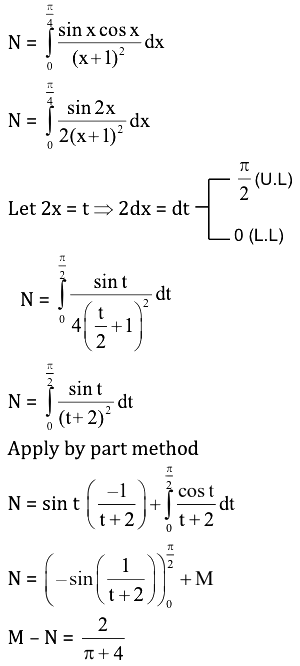Question 37: The value of the integral I = ∫1/20142014 [tan-1 x/x] dx is

1. a. (𝛑/4) log 2014
2. b. (𝛑/2) log 2014
3. c. 𝛑 log 2014
4. d. (1/2) log 2014

Solution:

I = ∫1/20142014 [tan-1 x/x] dx ---- (1)

Let x = 1 / t

dx = [-1/t2] dt

I = ∫20141/2014 [[tan-1 (1/t)] / [1/t]} dt

I = ∫1/20142014 [cot-1 t / t] dt --- (2)

From eq(1) + eq(2)

2I = ∫1/20142014 {[tan-1 t + cot-1 t] / t} dt

2I = ∫1/20142014 [(𝛑 / 2) / t] dt

I = (𝛑 / 4) (ln t)1/20142014

I = (𝛑 / 4) [ln 2014 - ln (1 / 2014)]

= (𝛑 / 4) [2 ln 2014]

= (𝛑 / 2) log 2014

Question 38: Let I = ∫𝛑/4𝛑/3 [sinx / x] dx, then

1. a. (1/2) ≤ I ≤ 1
2. b. 4 ≤ I ≤ 2√30
3. c. √3/8 ≤ I ≤ √2/6
4. d. 1 ≤ I ≤ 2√3/√2

Solution:

I = ∫𝛑/4𝛑/3 [sinx / x] dx

(sin x / x) is a decreasing function

So [(𝛑 / 12) * (sin (𝛑 / 3)) / (𝛑 / 3)] ≤ I ≤ [(𝛑 / 12) * (sin (𝛑 / 4)) / (𝛑 / 4)]

(1 / 4) * (√3 / 2) ≤ I ≤ (1 / 3) * (1 / √2)

√3/8 ≤ I ≤ √2/6

Question 39: The value of I =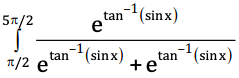dx, is

1. a. 1
2. b. 𝛑
3. c. e
4. d. 𝛑 / 2

Solution: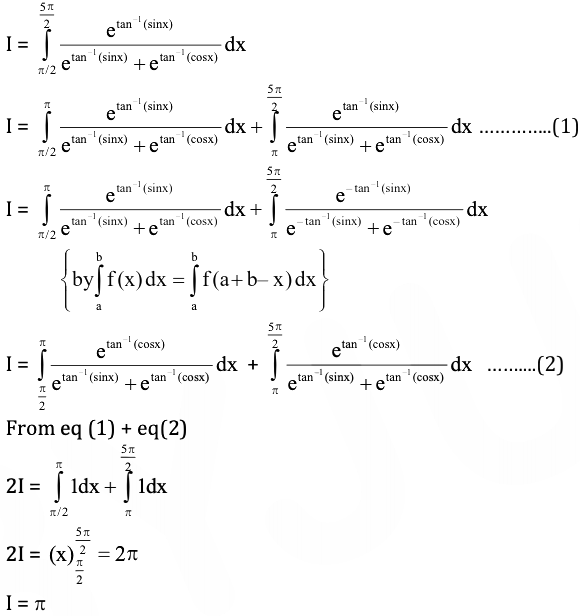Question 40: The value of limn→∞ (1/n) {sec2 (𝛑 / 4n) + sec2 (2𝛑 / 4n) + …. + sec2 (n𝛑 / 4n)} is

1. a. logc2
2. b. 𝛑 / 2
3. c. 4 / 𝛑
4. d. e

Solution:

L = limn→∞ {sec2 (𝛑 / 4n) + sec2 (2𝛑 / 4n) + …. + sec2 (n𝛑 / 4n)}

L = limn→∞ (1/n) ∑r=1n sec2 (r𝛑 / 4n)

Let (r / n) = x

(1 / n) = dx

L = ∫01 sec2 (𝛑x/4) dx

= [tan (𝛑x / 4)]01 * (4/𝛑)

= (4/𝛑) (tan [𝛑/4] - tan 0])

= 4/𝛑

Question 41: The differential equation representing the family of curves y2 = 2d (x + √d) where d is a parameter, is of

1. a. order 2
2. b. degree 2
3. c. degree 3
4. d. degree 4

Solution:

y2 = 2d (x + √d) ---- (i)

Differentiate with respect to x

2y (dy / dx) = 2d

d = y (dy / dx)

Put in equation (i)

y2 = 2y (dy/dx) [x + √y (dy / dx)]

y2 = 2xy (dy/dx) + 2y3/2 (dy / dx)3/2

(y2 - 2xy [dy/dx])2 = 4y3 (dy / dx)3

Degree three.

Question 42: Let y(x) be a solution of (1 + x2) (dy / dx) + 2xy – 4x2 = 0 and y(0) = –1. Then y(1) is equal to

1. a. 1/2
2. b. 1/3
3. c. 1/6
4. d. –1

Solution:

(1 + x2) (dy / dx) + 2xy – 4x2 = 0

(dy / dx) + (2x / [1 + x2]) * y = 4x2 / (1 + x2)

IF = e∫Pdx = e∫(2x/1+x2) dx = eln(1+x2) = (1 + x2)

y (1 + x2) = ∫[(4x2) / (1 + x2)] * (1 + x2) dx + c

y (1 + x2) = ∫4x2 dx + c

y (1 + x2) = (4x3 / 3) + c

Put y(0) = –1

–1 = c

y (1 + x2) = (4x3 / 3) - 1

y(1) = y (1 + 1) = (4 / 3) - 1

2y = 1/3

y = 1/6

Question 43: The law of motion of a body moving along a straight line is x = (1/2) vt, x being its distance from a fixed point on the line at time t and v is its velocity there. Then

1. a. acceleration f varies directly with x
2. b. acceleration f varies inversely with x
3. c. acceleration f is constant
4. d. acceleration f varies directly with t

Solution:

x = (1 / 2) vt

Differentiate with respect to x

x = (1 / 2) (dx / dt) * t

∫2dt / t = ∫dx / x

ln c + 2 ln t = ln x

x = t2c

(dx / dt) = 2 tc

d2x / dt2 = 2c

Hence, acceleration is constant.

Question 44: Number of common tangents of y = x2 and y = –x2 + 4x – 4 is

1. a. 1
2. b. 2
3. c. 3
4. d. 4

Solution: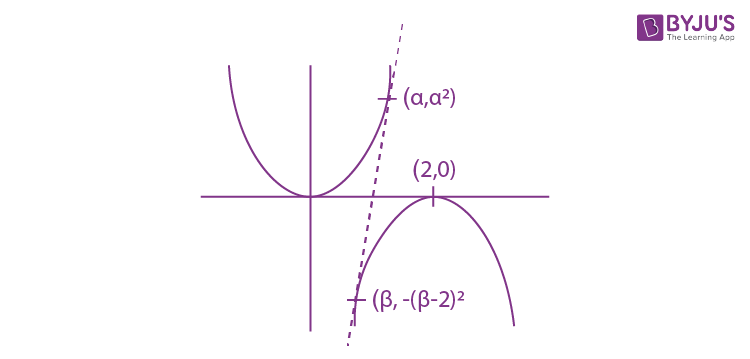y = x2; y = -(x - 2)2

2 + (β - 2)2] / [α - β] = 2α = -2 [β - 2]

α = 2 - β

β = 2 – α

2 + α2] / [α - 2 + α] = 2α

[2α2 / 2α - 2] = 2α

α2 = α (2α - 2)

α2 = 2α2 - 2α

α2 = 2α

α = 0, 2

α = 0, β = 2

α = 2, β = 0

Hence, two common tangents.

Question 45: Given that n numbers of A.Ms are inserted between two sets of numbers a, 2b and 2a, b where a, b ∈ R. Suppose further that the mth means between these sets of numbers are same, then the ratio a : b equals

1. a. n – m + 1 : m
2. b. n – m + 1 : n
3. c. n : n – m + 1
4. d. m : n – m + 1

Solution:

a..........n A.Ms...........2b

(Difference)d = (2b - a) / (n + 1)

Am = (a + m) ((2b - a) / (n + 1)) ---- (i)

2a..........n A.Ms...........b

d = (b - 2a) / (n + 1)

Am = 2a + m ((b - 2a) / (n + 1)) --- (ii)

Equating equation (i) & (ii)

a = [m / (n + 1)] (b + a)

(a / b) = m / [n - m + 1]

Question 46: If x + log10(1 + 2x) = x log105 + log106 then the value of x is

1. a. 1 / 2
2. b. 1 / 3
3. c. 1
4. d. 2

Solution:

x + log10(1 + 2x) = x log105 + log106

x log1010 + log10(1 + 2x) = log105x + log106

log10(1 + 2x) = log105x + log106 – log10 10x

log10(1 + 2x) = log10 [5x . 6] / [10x]

1 + 2x = [6 . 5x] / [2x . 5x]

1 + 2x = 6 / 2x

let 2x = t

1 + t = 6 / t

t2 + t – 6 = 0

(t + 3)(t – 2) = 0

t = –3(not possible)

2x = –3

t = 2

2x = 2

x = 1

Question 47: If Zr = sin (2𝛑r / 11) - i cos (2𝛑r / 11) then ∑r=010 Zr =

1. a. –1
2. b. 0
3. c. i
4. d. –i

Solution:

Given

Zr = sin (2𝛑r / 11) - i cos (2𝛑r / 11)

Zr = -i [cos (2𝛑r / 11) + i sin (2𝛑r / 11)]

Zr = -iei(2𝛑r / 11)

Now,

r=010 Zr = -i ∑r=010 ei(2𝛑r / 11)

= –i(0)

= 0

Question 48: If z1 and z2 be two non zero complex numbers such that (z1 / z2) + (z2 / z1) = 1, then the origin and the points represented by z1 and z2

1. a. lie on a straight line
2. b. form a right-angled triangle
3. c. form an equilateral triangle
4. d. form an isosceles triangle

Solution: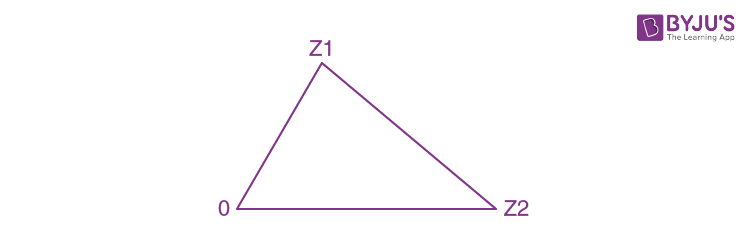z1 = z2ei𝛑/3

2z1 = z2 (1 + i√3)

2z1 = z2 + i√3z2

2z1 – z2 = i√3z2

Squaring both side

4z12 + z22 – 4z1z2 = -3z22

4z12 + z22 = 4z1z2

Hence from equilateral triangle.

Question 49: If b1b2 = 2(c1 + c2) and b1, b2, c1, c2 are all real numbers, then at least one of the equations. x2 + b1x + c1 = 0 and x2 + b2x + c2 = 0 has

1. a. real roots
2. b. purely imaginary roots
3. c. roots of the form a + ib (a, b ∈ R, ab ≠ 0)
4. d. rational roots

Solution:

Suppose the equations x2 + b1x + c1 = 0 & x2 + b2x + c2 = 0 have real roots.

then

b12 ≥ 4c1 .....(i)

b22 ≥ 4c2 .....(ii)

Given that b1b2 = 2(c1 + c2)

On squaring

b12b22 = 4 [c12 + c22 + 2c1c2] = 4 [(c1 - c2)2 + 4c1c2]

b12b22 - 16c1c2 = 4 [(c1 - c2)2] ≥ 0

Multiplying (i) & (ii), we get

b12b22 ≥ 16c1c2

Therefore, at least one equation has real roots.

Question 50: The number of selection of n objects from 2n objects of which n are identical and the rest are different is

1. a. 2n
2. b. 2n – 1
3. c. 2n – 1
4. d. 2n –1 + 1

Solution:

Total no. of ways = nC0 + nC1 + nC2 + ..........+ nCn

= 2n

Question 51: Let A be the centre of the circle x2 + y2 – 2x – 4y – 20 = 0. Let B(1, 7) and D(4, – 2) be two points on the circle such that tangents at B and D meet at C. The area of the quadrilateral ABCD is

1. a. 150 sq. units
2. b. 50 sq. units
3. c. 75 sq. units
4. d. 70 sq. units

Solution: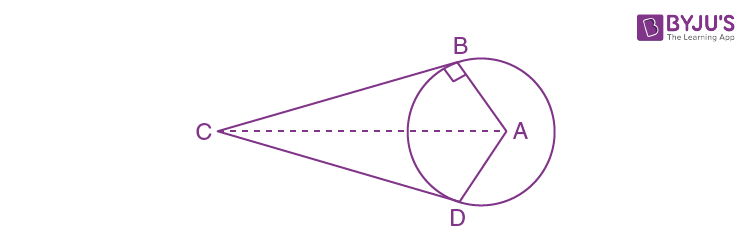s : x2 + y2 – 2x – 4y – 20 = 0

Centre of circle A = (1, 2)

Equation of tangent at B(1, 7)

x + 7y – (x + 1) –2 (y + 7) – 20 = 0

5y = 35

y = 7

Equation of tangent at D(4, –2)

4x – 2y – (x + 4) – 2(y – 2) – 20 = 0

3x – 4y = 20

coordinates of C are (16, 7)

Length of AB = √(1 - 1)2 + (7 - 2)2 = 5

Length of BC = √(16 - 1)2 + (7 - 7)2 = 15

The area of quadrilateral ABCD = 2 * (1 / 2) * 5 * 15

= 75 sq. units.

Question 52: Let f (x) =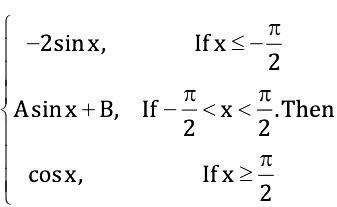1. a. f is discontinuous for all A and B
2. b. f is continuous for all A = – 1 and B = 1
3. c. f is continuous for all A = 1 and B = –1
4. d. f is continuous for all real values of A, B

Solution:

From the given conditions, function f (x) is continuous throughout the real line, when function f(x) is continuous at x = – 𝛑/2 and 𝛑/2 for continuity at x = – 𝛑/2.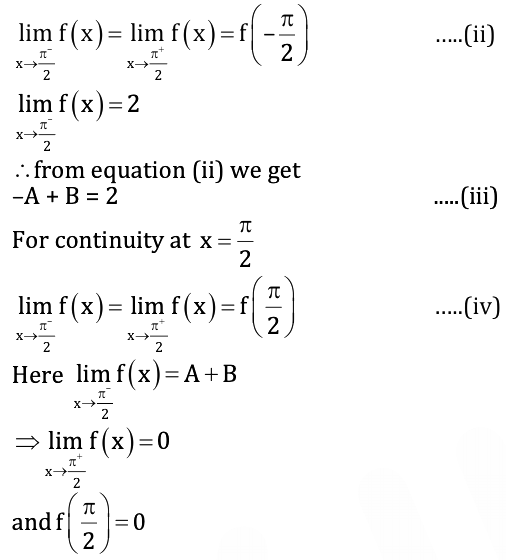from equation (iv)

A + B = 0 …..(v)

From equation (iii) & (iv)

A = –1, B = 1

Question 53: The normal to the curve y = x2 - x + 1, drawn at the points with the abscissa, x1 = 0, x2 = −1 and x3 = 5/2

1. a. are parallel to each other
2. b. are pairwise perpendicular
3. c. are concurrent
4. d. are not concurrent

Solution:

y = x2 - x + 1

(dy / dx) = 2x - 1 = mT

mN = -1 / mT

mN = 1 / (1 - 2x)

For tangent

mx1 = 1 Point (0, 1)

(y – 1) = 1(x – 0)

x – y + 1 = 0 …..(i)

For tangent

mx2 = (1 / 3) point (-1, 3)

(y – 3) = (1 / 3) (x + 1)

3y – 9 = x + 1

x – 3y + 10 = 0 …..(ii)

For normal

mx3 = (-1 / 4) point (5 / 2, 19 / 4)

(y - [19 / 4]) = - 1 / 4 [x - (5 / 2)]

x + 4y = 43 / 2 ---- (iii)

To find intersection point tangent (1) & tangent (2)

y – 1 – 3y + 10 = 0

–2y = –9

y = 9 / 2

Intersection point is (7 / 2, 9 / 2) passes (3)

Hence, normal are concurrent.

Question 54: The equation x log x = 3 – x

1. a. has no root in (1, 3)
2. b. has exactly one root in (1, 3 )
3. c. x log x – (3 – x) > 0 in [1, 3]
4. d. x log x – (3 – x) < 0 in [1, 3]

Solution:

f (x) = x log x – 3 + x

Differentiate with respect to x

f ‘ (x) = x * (1 / x) + log x + 1

f’ (x) = 2 + log xf(1)f(3) = –2 (3log3) = –ve

Hence, one root is (1, 3).

Question 55: Consider the parabola y2 = 4x. Let P and Q be points on the parabola where P (4, –4) & Q (9, 6). Let R be a point on the arc of the parabola between P & Q. Then the area of ΔPQR is largest when

1. a. ∠PQR = 90°
2. b. R(4, 4)
3. c. R (1/4, 1)
4. d. R (1, 1/4)

Solution: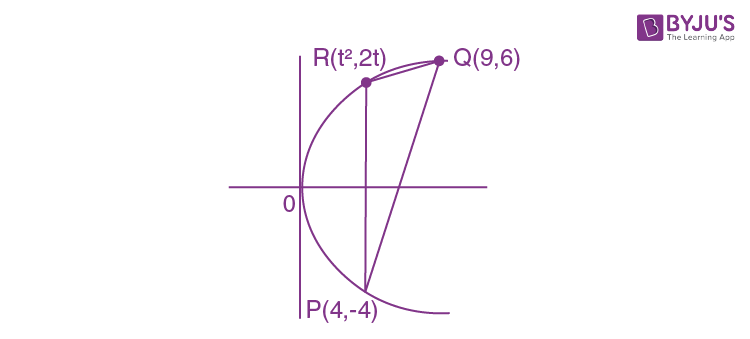Area = (1/2)

$$\begin{vmatrix} t^2 & 2t &1 \\ 9 & 6 & 1\\ 4 &-4 &1 \end{vmatrix}$$

= (1/2) [t2 (10) - 2t (5) + 1 (-60)]

= (10/2) (t2 - t - 6)

f (t) = 5t2 – 5t – 30

f’(t) = 10t – 5 = 0

t = 1 / 2

Point R = [(1/2)2, 2 (1/2)] = (1/4, 1)

Question 56: A ladder 20 ft long leans against a vertical wall. The top-end slides downwards at the rate of 2 ft per second. The rate at which the lower end moves on a horizontal floor when it is 12 ft from the wall is

1. a. 8/3
2. b. 6/5
3. c. 3/2
4. d. 17/4

Solution: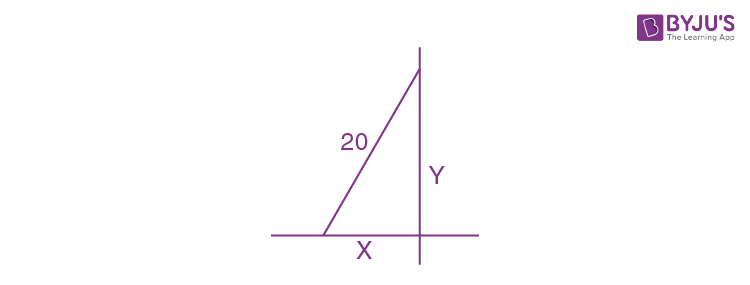Using right angle triangle concept

x2 + y2 = 400

Differentiate with respect to t

2x (dx / dt) + 2y (dy / dt) = 0

Given

(dy / dt) = 2ft / sec

x = 12, y= 16

2 (12) (dx / dt) + 2 (16) (dy / dt) = 0

(dx / dt) = -8/3

Question 57: For 0 ≤ p ≤ 1 and for any positive a, b; let I(p) = (a + b)P, J(p) = aP + bP, then

1. a. I(p) > J(p)
2. b. I(p) < J(p)
3. c. I(p) < J(p) in [0, p/2] and I(p) > J(p) in [p/2, ∞)
4. d. I(p) < J(p) in [p/2, ∞) and J(p) < I(p) in [0, p/2)

Solution:

a = 9

b = 16

I(P) = 5 and J(P) = 7

J(P) > I(P)

Now,

a = 1 / 9 and b = 1 / 16

I(P) = 5 / 12 & J(P) = 7 / 12

J(P) > I(P)

Question 58: Let α = i + j + k, β = i - j - k and γ = -i + j - k be three vectors. A vector δ, in the plane of α and β, projection on γ is 1 / √3, is given by

1. a. -i - 3j - 3k
2. b. i - 3j - 3k
3. c. -i + 3j + 3k
4. d. i + 3j - 3k

Solution:

Given that

α = i + j + k

β = i - j - k

γ = -i + j - k

γ = √3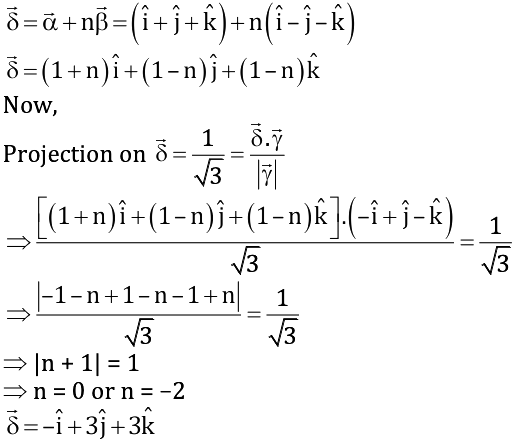Question 59: Let α, β, γ be three unit vectors such that α . β = α . γ and the angle between β and γ and is 30°. Then α is

1. a. 2 (β × γ)
2. b. − 2(β × γ)
3. c. ± 2(β × γ)
4. d. (β × γ)

Solution:

α . β = α . γ

Thus, α is perpendicular to b and c.

A unit vector perpendicular to b and c

= ± (β × γ) / |(β × γ)|

= ± (β × γ) / [|β| |γ| sin (𝛑 / 6)]

= ± (β × γ) / (1 / 2)

= ± 2 (β × γ)

Question 60: Let z1 and z2 be complex numbers such that z1 ≠ z2 and |z1| = |z2|. If Re(z1) > 0 and Im(z2) < 0, then [z1 + z2] / [z1 - z2] is

1. a. one
2. b. real and positive
3. c. real and negative
4. d. purely imaginary

Solution:

z1 = x1 + iy1 and z2 = x2 + iy2

Re(z1) > 0 ⇒ x1 > 0 and Im(z2) < 0 ⇒ y2 < 0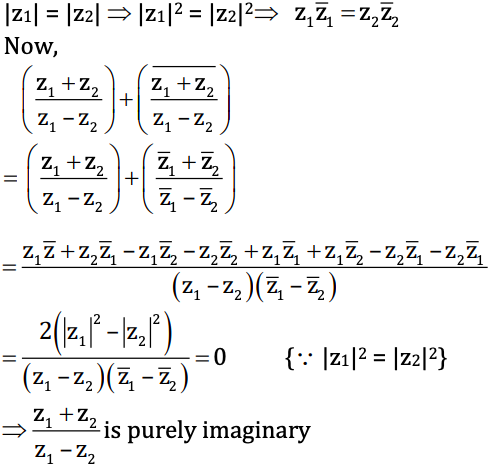Question 61: From a collection of 20 consecutive natural numbers, four are selected such that they are not consecutive. The number of such selections is

1. a. 284 × 17
2. b. 285 × 17
3. c. 284 × 16
4. d. 285 × 16

Solution:

1, 2, 3, 4, 5, ..........., 19, 20

There are 17 ways for four consecutive number

Number ways = 20C4 – 17

= [20 × 19 × 18 × 17] / [1 × 2 × 3 × 4] –17

= [285 × 17] – 17

= 284 × 17

Question 62: The least positive integer n such that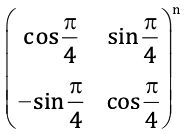is an identity matrix of order 2 is

1. a. 4
2. b. 8
3. c. 12
4. d. 16

Solution: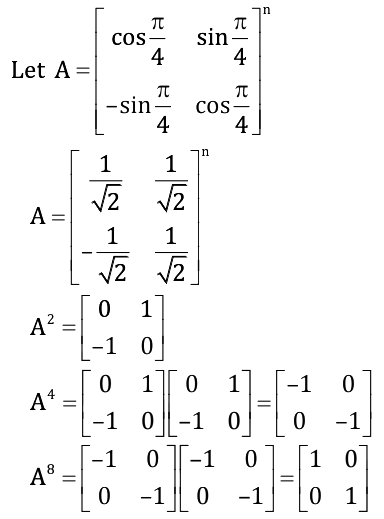Question 63: Let 𝛒 be a relation defined on N, the set of natural numbers, as 𝛒 = {(x, y) ∈ N × N : 2x + y = 41} Then

1. a. is an equivalence relation
2. b. is only reflexive relation
3. c. is only symmetric relation
4. d. is not transitive

Solution:

P = {(x, y) N × N : 2x + y = 41}

For reflexive relation

⇒ xRx ⇒ 2x + x = 41 ⇒ x = 41 / 3 ∉ N (not reflexive)

For symmetric

⇒xRy ⇒ 2x + y = 41 ≠ yRx (not symmetric)

For transitive

⇒ xRy ⇒ 2x + y = 41 and yRz ⇒ 2y + z = 41 x R z (not transitive)

Question 64: If the polynomial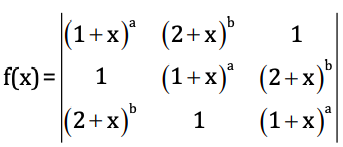then the constant term of f(x) is

1. a. 2 – 3.2b + 23b
2. b. 2 + 3.2b + 23b
3. c. 2 + 3.2b – 23b
4. d. 2 – 3.2b – 23b

Solution: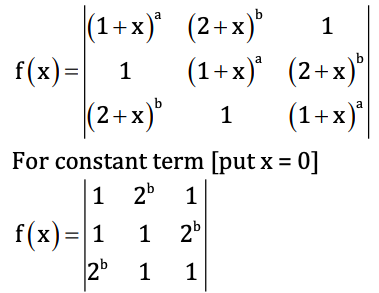f(x) = 1(1 – 2b) – 2b(1 – 22b) + 1(1 – 2b)

f(x) = 1 – 2b – 2b + 23b + 1 – 2b

f(x) = 2 – 3.2b + 23b

Question 65: A line cuts the x-axis at A(5, 0) and the y-axis at B(0, –3). A variable line PQ is drawn perpendicular to AB cutting the x-axis at P and the y-axis at Q. If AQ and BP meet at R, then the locus of R is

1. a. x2 + y2 – 5x + 3y = 0
2. b. x2 + y2 + 5x + 3y = 0
3. c. x2 + y2 + 5x – 3y = 0
4. d. x2 + y2– 5x – 3y = 0

Solution: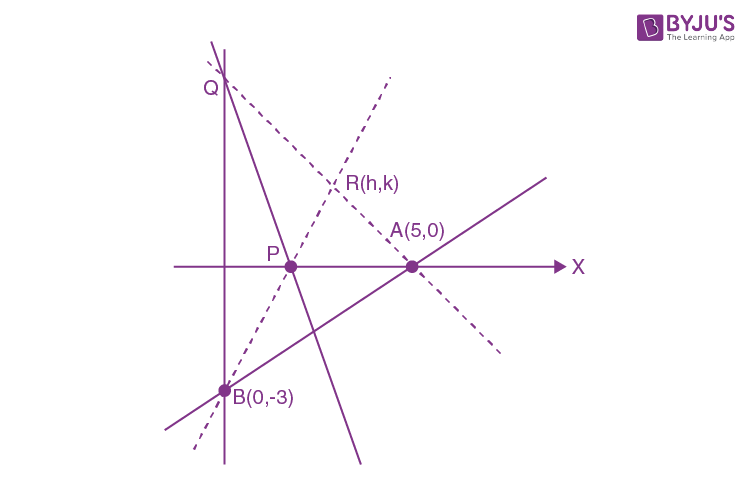Line AB is (x / 5) + (y / -3) = 1 ⇒ 3x – 5y = 15

Any perpendicular line to AB

5x + 3y = λ

So, P (λ / 5, 0), Q (0, λ / 3)

AQ is (x / 5) + y / (λ / 3) = 1

(3y / λ) = 1 - (x / 5)

(1 / λ) = (1 / 3y) (1 - (x / 5)) ---- (i)

And BP is x / (λ / 5) - (y / 3) = 1

5x / λ = 1 + (y / 3)

1 / λ = (1 / 5x) (1 + [y / 3]) --- (ii)

Equation (i) = equation (ii)

(1 / 3y) (1 - (x / 5)) = (1 / 5x) (1 + [y / 3])

5x (1 - [x / 5]) = (1 / 5x) (1 + [y / 3])

5x – x2 = 3y + y2

x2 + y2 – 5x + 3y = 0

Question 66: In a third order matrix A, aij denotes the element in the i-th row and j-th column. If aij = 0 for i = j

= 1 for i > j

= –1 for i < j Then the matrix is

1. a. skew-symmetric
2. b. symmetric
3. c. not invertible
4. d. non-singular

Solution:

Given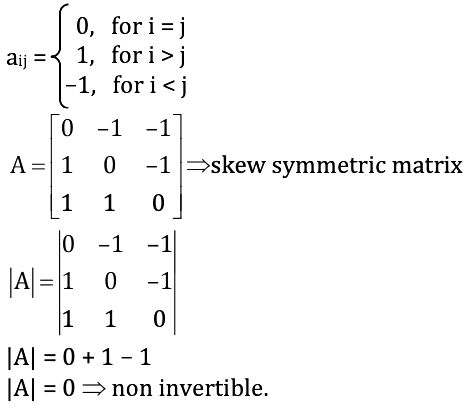Question 67: The area of the triangle formed by the intersection of a line parallel to the x-axis and passing through P(h, k), with the lines y = x and x + y = 2 is h2. The locus of the point P is

1. a. x = y – 1
2. b. x = –(y – 1)
3. c. x = 1 + y
4. d. x = –(1 + y)

Solution: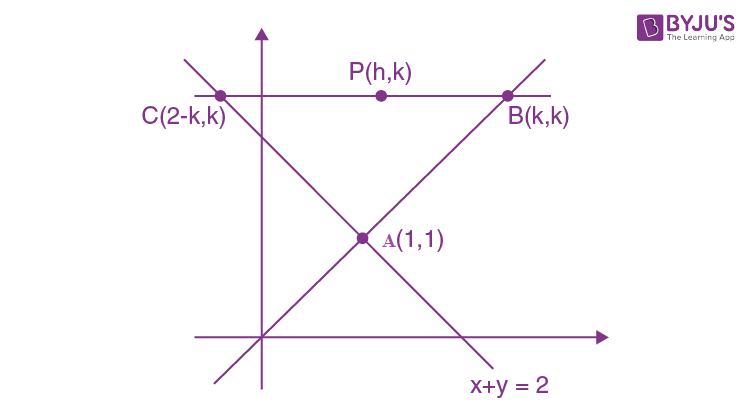Area of triangle = h2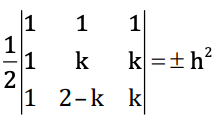1(k2 – (2 – k)k) – 1(k – k) + 1(2 – k – k) = ± 2h2

k2 – 2k + k2 + 2 – 2k = ± 2h2

2k2 – 4k + 2 = ± 2h2

k2 – 2k + 1 = ± h2

Locus is (k – 1)2 = h2

y – 1 = ± x

x – y + 1 = 0 or x + y = 1

x = y – 1 or x = –(y – 1)

Question 68: A hyperbola, having the transverse axis of length 2 sin θ is confocal with the ellipse 3x2 + 4y2 = 12. Its equation is

1. a. x2 sin2 θ – y2 cos2 θ = 1
2. b. x2 cosec2 θ – y2 sec2 θ = 1
3. c. (x2 + y2) sin2 θ = 1 + y2
4. d. x2 cosec2 θ = x2 + y2 + sin2 θ

Solution:

The length of transverse axis = 2sin θ = 2a

a = sin θ

Also for ellipse, 3x2 + 4y2 = 12

i.e. (x2 / 4) + (y2 / 3) = 1

a2 = 4 & b2 = 3

Now,

e = √1 - [b2 / a2]

e = √1 - (3 / 4) = ± (1 / 2)

focus of ellipse is = (ae, 0) & (–ae, 0)

focus = (1, 0) and (–1, 0)

As the hyperbola if confocal focus is same

And length of transverse axis = 2sin θ

length of semi transverse axis = sin θ

i.e. A = sin θ

And C = 1 where A, B, C are parameters in hyperbola similar to ellipse

C2 = A2 + B2

B2 = 1 – sin2 θ = cos2 θ

Equation of hyperbola is (x2 / A2) - (y2 / B2) = 1

x2 / sin2 θ – y2 / cos2 θ = 1

x2 cosec2 θ – y2 sec2 θ = 1

Question 69: Let f (x) = cos (𝛑 / x), x ≠ 0, then assuming k as an integer,

1. a. f (x) increases in the interval [1 / (2k + 1), 1 / 2k]
2. b. f (x) decreases in the interval [1 / (2k + 1), 1 / 2k]
3. c. f (x) decreases in the interval [1 / (2k + 2), 1 / 2k + 1]
4. d. f (x) increases in the interval [1 / (2k + 2), 1 / 2k + 1]

Solution:

f (x) = cos (𝛑 / x)

Differentiate with respect to x

f ‘ (x) = - sin (𝛑 / x) . (-𝛑 / x2)

= (𝛑 / x2) sin (𝛑 / x) > 0

For increasing function f’(x) > 0

sin (𝛑 / x) > 0

(2k𝛑) < 𝛑 / x < (2k + 1) 𝛑

(1 / 2k) > x > (1 / [2k + 1])

For decreasing function f’(x) < 0

sin (𝛑 / x) < 0

(𝛑 / x) ∈ [(2k + 1) 𝛑, (2k + 2) 𝛑]

(𝛑 / x) ∈ [1 / (2k + 2), 1 / 2k + 1]

Question 70: Consider the function y = loga (x + √x2 + 1), a > 0, a ≠ 1. The inverse of the function

1. a. does not exist
2. b. is x = log1/a (y + √y2 + 1)
3. c. is x = sinh (y ln a)
4. d. is x = cosh (-y ln (1 / a))

Solution: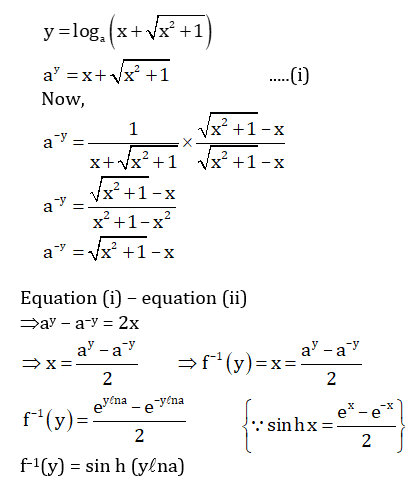Question 71: Let I = ∫01 [x3 cos 3x] / [2 + x2] dx, then

1. a. (-1 / 2) < I < (1 / 2)
2. b. (-1 / 3) < I < (1 / 3)
3. c. – 1 < I < 1
4. d. (-3 / 2) < I < (3 / 2)

Solution:

1. Answer: (a, b, c, d)

We know that

–1 < cos 3x < 1

–x3 < x3cos 3x < x3

-x3 / [2 + x2] < [x3 cos 3x] / [2 + x2] < x3 / [2 + x2]

Taking integration from 0 to 1

01 -x2 dx < 1 < ∫01 x2 dx

[-x3 / 3]01 < I < (x3 / 3)01

(-1 / 3) < I < (1 / 3)

Question 72: A particle is in motion along a curve 12y – x3. The rate of change of its ordinate exceeds that of abscissa in

1. a. – 2 < x < 2
2. b. x = ± 2
3. c. x < – 2
4. d. x > 2

Solution:

Given

(dy / dt) > (dx / dt) .....(i)

12y = x3

Differentiate with respect to

12 (dy / dt) = 3x2 (dx / dt) .....(ii)

From equation (i)

3x2 (dx / dt) > 12 (dx / dt)

x2 – 4 > 0

x ∈ (–∞, –2) ⋃ (2, ∞)

Question 73: The area of the region lying above x-axis, and included between the circle x2 + y2 = 2ax & the parabola y2 = ax, a > 0 is

1. a. 8𝛑a2
2. b. a2 [(𝛑 / 4) - (2 / 3)]
3. c. 16𝛑a2 / 9
4. d. 𝛑 [(27 / 8) + 3a2]

Solution:

Given

C1: x2 + y2 = 2ax

C2: y2 = ax

To find intersection points

x2 + (ax) = 2ax

x2 = ax

x(x – a) = 0

x = 0, a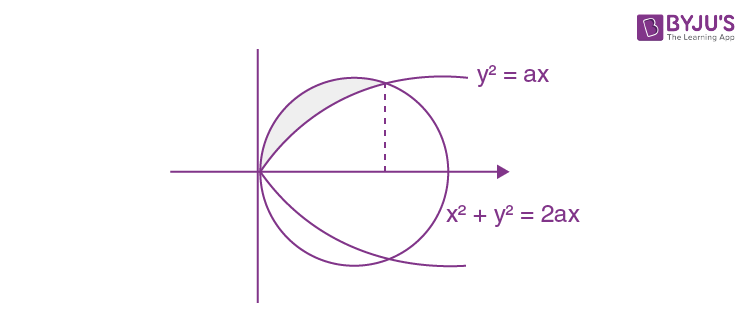Area = (1 / 4) (area of circle) - ∫0a √ax dx

= (1 / 4) 𝛑a2 - √a [(x3/2 / [3 / 2]]0a

= (𝛑a2 / 4) - (2a2 / 3)

= a2 [(𝛑 / 4) - (2 / 3)]

Question 74: If the equation x2 – cx + d = 0 has roots equal to the fourth powers of the roots of x2 + ax + b = 0, where a2 > 4b, then the roots of x2 – 4bx + 2b2 – c = 0 will be

1. a. both real
2. b. both negative
3. c. both positive
4. d. one positive and one negative

Solution:

Let x2 + ax + b = 0 has roots α and β

x2 – cx + d = 0 roots are α4 and β4

α + β = –a .....(i)

α β = b .....(ii)

α4 + β4 = c .....(iii)

(α β)4 = d .....(iv)

From equation (ii) & (iv)

b4 = d

And α4 + β4 = c

2 + β2)2 - 2(αβ)2 = c

((α + β)2 - 2(αβ))2 - 2(αβ)2 = c

(a2 – 2b)2 – 2b2 = c

2b2 + c = (a2 – 2b)2

Now for equation x2 – 4bx + 2b2 – c = 0

D = (4b)2 – 4(1)(2b2 – c)

D = 16b2 – 8b2 + 4c

D = 4(2b2 + c)

D = 4(a2 – 2b)2 > 0 ⇒ real roots

Now,

f(0) = 2b2 – c

f(0) = a2(4b – a2) < 0 {since a2 > 4b}

Roots are opposite in sign.

Question 75: On the occasion of Diwali festival each student of a class sends greeting cards to others. If there are 20 students in the class, the number of cards sent by students are

1. a. 20C2
2. b. 20P2
3. c. 2 * 20C2
4. d. 2 * 20P2

Solution:

Total students = 20

Number of ways = 20C2 × 21

= {[20 * 19] / 2} * 2

= 20 × 19

= 20P2

### WBJEE 2018 Maths Question Paper with Solutions